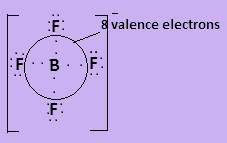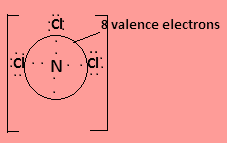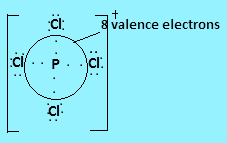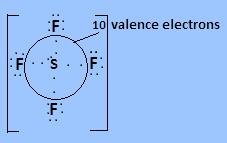Courses
Courses for Kids
Free study material
Free LIVE classes
MoreLIVE
Join Vedantu’s FREE Mastercalss

# In which of the following species central atoms are not surrounded by exactly $8$ valence electrons?A)${\text{BF}}_4^ -$ B)${\text{NC}}{{\text{l}}_3}$ C)${\text{PCl}}_4^ +$ D)${\text{S}}{{\text{F}}_4}$Verified
335.1k+ views
Hint:
The valence electrons are the electrons present in the outermost shell so they easily participate in chemical bonding. In covalent bond, both the atoms of the molecule contribute one valence electron to form a bond.

Here all given molecules have covalent bonds present in them. In ${\text{BF}}_4^ -$, boron has three valence electrons and each fluorine atom contributes one valence electron to form covalent bond. The negative charge on the molecule contributes one more valence electron so the species contain $8$ valence electrons. The Lewis structure is-In ${\text{NC}}{{\text{l}}_3}$nitrogen has three valence electrons and one lone pair electron and each chlorine contributes one valence electrons to form covalent bond so the central atom is surrounded by $8$ valence electrons. The Lewis structure is-In ${\text{PCl}}_4^ +$ phosphorus has five valence electrons, four of which will form covalent bonds with four chlorine atoms and the positive charge on the molecule indicates loss of one electron. So the central atom is surrounded by $8$ valence electrons.In ${\text{S}}{{\text{F}}_4}$ Sulphur has six valence electrons in which four valence electrons bond with each fluorine atom and two electrons are present as lone pair. So $10$ valence electrons are present around the central atom.So the correct answer is D.

Note:
Here the structures drawn are known as lewis structure or electron dot diagrams which show the electrons and lone pairs arranged around an atom in a molecule. Here, electrons are shown by dots. Here the bonded electrons can be joined by a line to show the bond but we have not drawn the line so that we can easily count the electrons around the central atom.

Last updated date: 28th Sep 2023
Total views: 335.1k
Views today: 5.35k